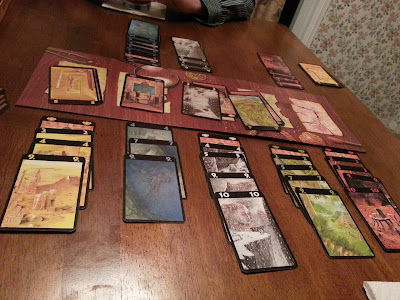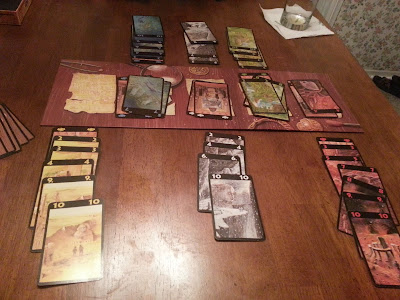Round 1 (20120804)

20090824:
I found an undated piece of paper with two games of Lost Cities between Daniel and me. I don't know if it was for this day, but instinct tells me it was.

Detailed breakdown:
G#R#I_-_1-_2-_3-_4-_5=-_T
0101DM-06+09+20+02+00=+25
0101TT+07-01+03+12+00=+21
0102DM-01+09+01-10+00=-01
0102TT+06-18+16+02+00=+06
0103DM+XX+XX+XX+XX+XX=+15
0103TT+04+48-04+21+00=+69

0201DM+01-14-03-18+00=-34
0201TT-04+36+12+20+00=+64
0202DM-21+06+01-08+00=-22
0202TT+38+08-08+04+00=+42
0203DM+08+08+00+08+00=+24
0203TT+17+06+06+00+00=+29
Notation: Game #; Round #; Initials; One of the colors; Cumulative TotalRound 2 (20120804)

20100122:
Whoa... I didn't write down what I got on the first game, but it was also a horrible loss. I wonder what the strategy is, because I'm pretty sure I don't know it.

20100417:
Caroline's first game of Lost Cities. In a game consisting of one round, had Caroline drawn from the board instead of the draw pile, she would have avoided losing 14 points for a total of 22 points, besting my score of 21 by 1 point.

Detailed breakdown:
G#R#I_-_Y-_B-_W-_G-_R=-_T
0001CB+09-20+05+14+00=+08
0001TT+01+00+00+05+15=+21
Alternative:
0001CB+09-06+05+14+00=+22

0101CB+04+06+05-24+00=-09
0101TT+00+00+15+17+18=+50
0102CB+10+14+07+00-16=+15
0102TT+00+14+58+00+01=+73
0103CB+34+16+00+00+00=+50
0103TT+00+00+71+03+07=+81
Notation: Game #; Round #; Initials; Yellow; Blue; White; Green; Red; Cumulative Total

[20100513 9:30 AM][20170526 Edit]

20120804:
On 20170526, I was going through various draft posts and eventually I came across one post with a bunch of numbers.

At first all I could tell was that Josh and I had played a game, but I couldn't make heads or tails of what game was being played. In attempting to solve the mystery I tried to figure out the differences between a lower-case 'x' and an upper-case 'X', but I didn't have much luck. Then, after studying the scores long enough, I had realized there were always five sets of scores per line. Somehow it then clicked to me that the scores were for a game of "Lost Cities"; I suppose the comment "[b]ad to have played all colors the way I did" had played its part in jogging my memory. However, in hindsight, I should have first tried using the draft's publish date (20120805) and cross-referencing that date against my picture log.

Detailed breakdown:
G#R#I_-_Y-_B-_W-_G-_R=-_T
0101JK+xx+62+01+xx+06=+69
0101TT-10+00+16+06+08=+20
0102JK+xx+22+01+09+xx=101
0102TT+16+xx+02+xx+44=+82
0103JK+00+24+18+32+03=178
0103TT+03+06-04-18-18=+51
Notation: Game #; Round #; Initials; Yellow; Blue; White; Green; Red; Cumulative Total.
Also 'xx' denotes the color was not played.



20090717 2P Josh Me. Josh won.
20090717 4p [Daniel Me] [Stephanie Josh]. Josh Me Stephanie Daniel. [Daniel Me] won.
20090824 2P Daniel Me.
20090919 2P Xuehua->Matt Me.
Several unlogged games...
20100122 2P [poortinytim] [jack]!.
20100122 2P [poortinytim] (22,-7,7) [kenklaw]!(106,36,40).
20100417 2P Caroline (8) Me!(21). Me first.
20100417 2P Caroline (-9,15,50) Me!(50,73,81). Me first.
20120804 2P Josh Me. Me first. Josh won.
Lost Cities